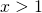# Solution assignment 10 Linear inequalities

### Assignment 10

Solve: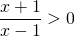### Solution

We draw three number lines: one for the numerator, the second one for the denominator and the third one for the quotient. The first line shows that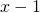is equal to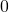for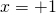, positivefor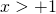and negative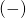for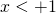.

----------------- (+1) ++++ x-1
----- (-1) +++++++++++ x+1
+++ (-1) ----- (+1) ++++ quotiënt

From this we can determine the sign of the quotient of both linear functions because:
- / - = +
+ / + = +
+ / - = -
- / - = +

and find: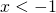or